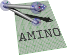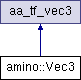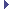amino  1.0-beta2 Lightweight Robot Utility Library
amino::Vec3 Struct Reference

A vector of length 3. More...

#include <tf.hpp>

Inheritance diagram for amino::Vec3:## Public Member Functions

Vec3 (const struct aa_tf_duqu *S)
Extract the translation part of a unit dual quaternion.

Vec3 (const struct aa_tf_duqu &S)
Extract the translation part of a unit dual quaternion.

Vec3 (const struct aa_tf_tfmat *T)
Extract the translation part of a transformation matrix.

Vec3 (const struct aa_tf_tfmat &T)
Extract the translation part of a transformation matrix.

Vec3 (double _x, double _y, double _z)
Construct from individual components.

Vec3 (const double *_xyz)
Construct from array.

## Static Public Member Functions

static aa_tf_vec3 from_xyz (double x, double y, double z)
Create a Vec3 from components.

static aa_tf_vec3 from_vec3 (const double a_x)
Create a Vec3 from array.

static aa_tf_vec3 from_duqu (const double S)
Create a Vec3 from translation part of unit dual quation.

static aa_tf_vec3 from_tfmat (const double T)
Create a Vec3 from translation part of transformation matrix.

## Additional Inherited MembersPublic Attributes inherited from aa_tf_vec3
union {
struct {
double   x
x component

double   y
y component

double   z
z component

}

double   data 
data array

};

## Detailed Description

A vector of length 3.

Definition at line 66 of file tf.hpp.

The documentation for this struct was generated from the following file: